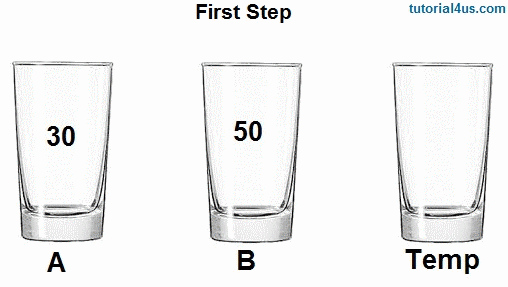Swap Two Numbers Without Using Third Variable

# Swap Two Numbers Without Using Third Variable

## Swapping of Two Numbers Program in C

Swap two numbers means exchange the values of two variables with each other. For example variable num1 contains 20 and num2 contains 40 after swap there values num1 contains 40 and num2 contains 20.

Example: Suppose we have three glass A, B, Temp. Glass A contain 30, Glass B contain 50 and Glass Temp is empty now we want to interchange these two glass (A, B) valuse. So we follow below steps...

• First we take Glass A and there values place inside Glass Temp
• Now Glass A is empty, take Glass B and there values place inside Glass A.
• Now Glass B is empty, take Glass Tempa and there values place inside Glass B
• Finall Both Glass values are exchange, Glass A contain 50 and Glass B contain 30.## Swap Two Numbers Program in C

```#include
#include

void main()
{
int a,b,c;
clrscr();
printf("Enter value of a: ");
scanf("%d",&a);
printf("Enter value of b: ");
scanf("%d",&b);
c=a;
a=b;
b=c;
printf("After swapping a=: %d b=: %d",a,b);
getch();```